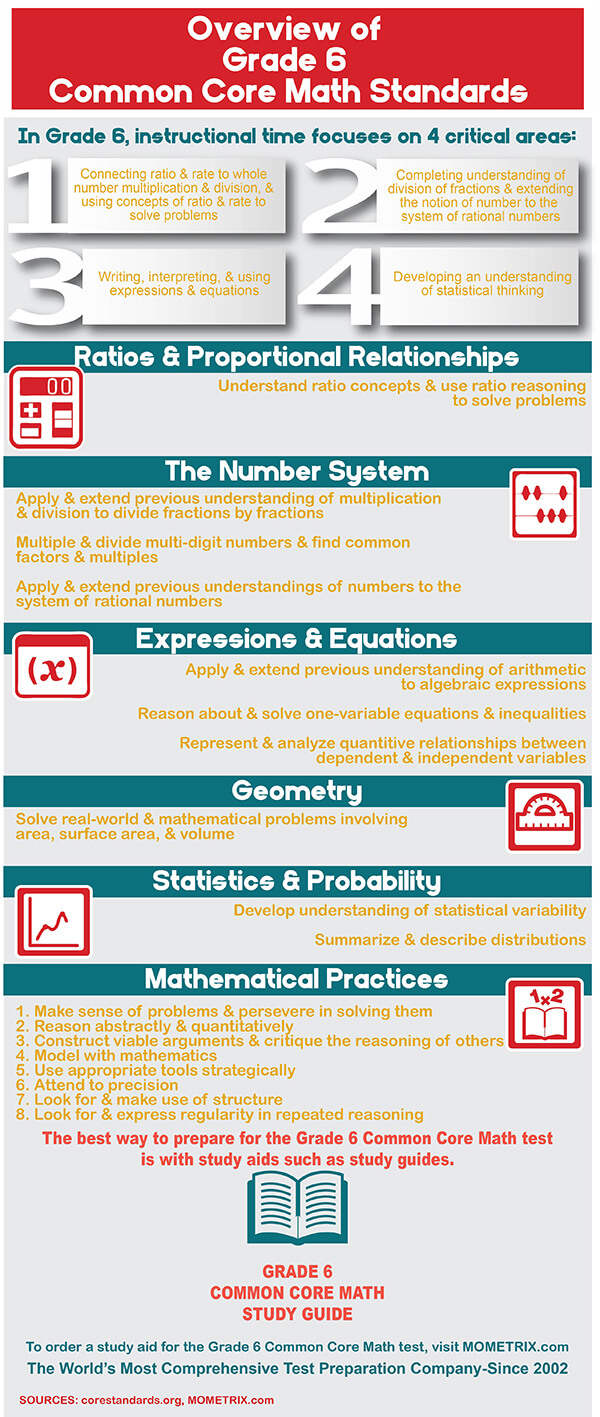# Mathematics Grade 6 Practice Questions

1. 1:1
2. 1:2
3. 2:½
4. 2:1
1. 10
2. 16
3. 20
4. 25
1. 16
2. 24
3. 48
4. 96
1. 60
2. 40
3. -40
4. -60
1. 2
2. 3
3. 6
4. 8
1. 2
2. 3
3. 4
4. 8
##### 7. Calculate the area of the right triangle below.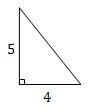1. 9
2. 10
3. 15
4. 20
1. 4
2. 8
3. 12
4. 16
##### 9. The test scores in Mr. Smith’s class are shown below.82, 94, 81, 70, 72, 78, 95, 83, 89, 80, 55, 77Create a histogram to display the data.
1.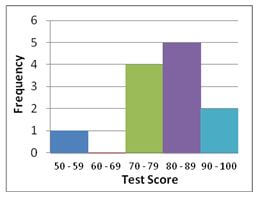2.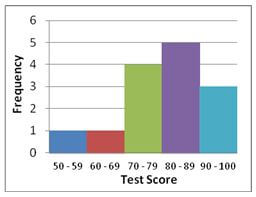3.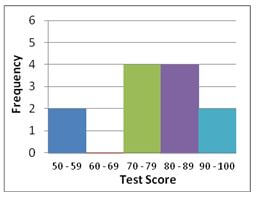4.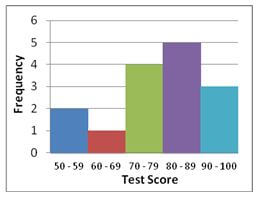##### 10. What unit of measure would be appropriate to use when investigating the attendance of nine different Broadway shows?
1. days
2. dollars
3. people
4. shows

1. D: A ratio is a relationship between two numbers of the same type. For instance, if the ratio of apples to oranges in a basket is 3:2, then the basket contains 3 apples for every 2 orange. In the given problem, we are looking for ratio between the populations of two cities. Since we are told that the population of New York is about twice that of Paris, there are about two New Yorkers for every Parisian. Therefore, the correct ratio is 2:1.

2. C: A percent represents one part out of 100. Thus, 40% is equivalent to 40 parts out of 100, or 40/100. To find 40% of 50, multiply 40/100 by 50:
40/100 X 50 = 20

3. B: The least common multiple (LCM) of two integers is the smallest (or least) integer that is a multiple of both numbers. One way to find the LCM of 8 and 12 is to list their multiples, and choose the smallest number that appears in both lists.

8: 8, 16, 24, 32, 40, 48
12: 12, 24, 36, 48, 60, 72

Since 24 is the smallest number in both lists, the LCM of 8 and 12 is 24.

4. D: An increase in the amount of your debt means you owe more than \$50. If, for instance, your debt increases by \$10, it becomes \$60 and your account balance becomes -60. Therefore, -60 could be your new balance. (Note that a balance of -40 means that you owe \$40, which would be a decrease in your debt.)

5. C: According to the order of operations (PEMDAS), evaluate any quantities or expressions in parentheses first. Subtract to find the value in the parentheses and then divide 24 by the result of that subtraction:

24 x (6 – 2) = 24 ÷ 4 = 6

6. A: A solution to an equation or inequality is a value of the variable(s) that makes the equation or inequality true. To determine which choice is a solution to the inequality 9 + 2x > 5x, substitute each one for x in the inequality and simplify the result to see if the inequality is true. Start with x = 2.

9 + 2x > 5x
9 + 2(2) > 5(2)
9 + 4 > 10
13 > 10

Since 13 > 10 is true, the correct answer is x = 2.
Alternatively, subtract 2x from both sides of the inequality to get 9 > 5x – 2x, which is equivalent to 9 > 3x. Divide both sides by 3 to get 3 > x, or x < 3. The only x to choose less than 3 is 2, so A is correct.

7. B: The area of a triangle is given by the formula A = ½bh , where b is the base of the triangle and h is the height of the triangle (drawn as a line perpendicular to the base). Since the triangle is a right triangle, you can use the horizontal leg for the base and the vertical leg for the height:

A = ½bh
= ½ (4)(5)
= ½ (20)
= 10

8. A: The mean of a data set is its average. Therefore, unless all of the values in the set are 12 (and you know they are not because the range is 8), some of them must be less than 12 and some must be more than 12.

The range of a data set is the difference between its largest and smallest values. Since the range of this set is 8, all values in the set have to be greater than 4. If one value was 4, there has to be a value greater than 12 to ensure the average stays 12, and the difference between that value and 4 would be more than 8. Since we know the range is 8, 4 could not be a value in the set.

9. A: A histogram is a way of graphically representing the distribution of a data set. In a histogram, the data range is divided into intervals so that every value falls in one interval. For the given test scores, one natural way to set the intervals is 50 – 59, 60 – 69, 70 – 79, 80 – 89, and 90 – 100.

Next calculate the number of values that fall within each interval, i.e. ask how many values are between 50 and 59 (including 50 and 59), how many are between 60 and 69, and so on: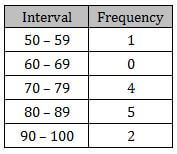Finally, display this information graphically using a vertical bar for each interval and making its height equal to the frequency of the interval:10. C: The appropriate unit of measure of a quantity corresponds to what you are counting when you measure it. For instance, if the price of a car is \$40,000, because you are counting dollars, the unit of measure of the quantity \$40,000 is dollars. To measure the attendance of the Broadway shows, you count the number of people who go to each show. Therefore, the unit of measure is people.